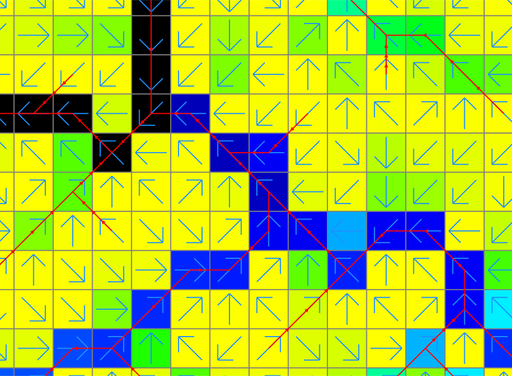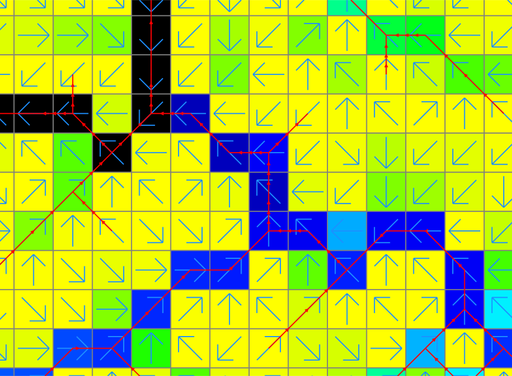Repository for Ideas & Research
Open Source GIS, Hydrologic Modeling, Optimization

# How to delineate stream networks in GRASS GIS

## 1   Identifying stream networks without calculating hydrologic parameters

Extract streams using the A* algorithm with a threshold value of 50,000 cells for stream generation:

``````# accumulation=flow_accum is optional
r.stream.extract elevation=elevation threshold=50,000 stream_vector=streams accumulation=flow_accum``````

Figure 1 shows a stream network vector map created using r.stream.extract. The stream vector properly represents flow directions.Figure 1: Stream networks generated using r.stream.extract. The red and blue lines represent the stream vector and flow directions, respectively. The underlying raster map is flow accumulation.

## 2   Delineating watersheds and identifying stream networks from the same source of elevation data

Calculate flow direction and accumulation, and delineate basins in the raster format:

``r.watershed -a elevation=elevation threshold=50,000 accumulation=flow_accum basin=basins``

Extract streams from the flow accumulation raster map from r.watershed so that the stream network output matches the watershed output from r.watershed:

``r.stream.extract elevation=elevation accumulation=flow_accum threshold=50,000 stream_vector=streams``

## 3   Delineating stream networks from a flow direction map

r.accumulate takes a flow direction map and delineates stream networks using a threshold:

``r.accumulate direction=drain_directions threshold=50000 stream=streams``

## 4   How not to delineate stream networks

r.watershed generates a stream raster map and you may be tempted to simply convert this stream raster map to vector to identify stream networks:

``````r.watershed -a elevation=elevation threshold=50,000 stream=streams
r.thin input=streams output=streams_thinned
r.to.vect input=streams_thinned output=streams type=line``````

However, there are two problems with this method. First, the output stream vector map does not guarantee the correct directionality of stream paths. Second, if there are raster cell clumps in the stream raster map, stream loops may be generated or even incorrect stream paths can be obtained. An example is shown in Figure 2. Note that the stream vector does not fully agree with flow directions. Compare this output to Figure 1.Figure 2: Stream networks generated using r.watershed, r.thin, and r.to.vect. The red and blue lines represent the stream vector and flow directions, respectively. The underlying raster map is flow accumulation.